# Hayes Process Macro - Model 4 - Mediation Analysis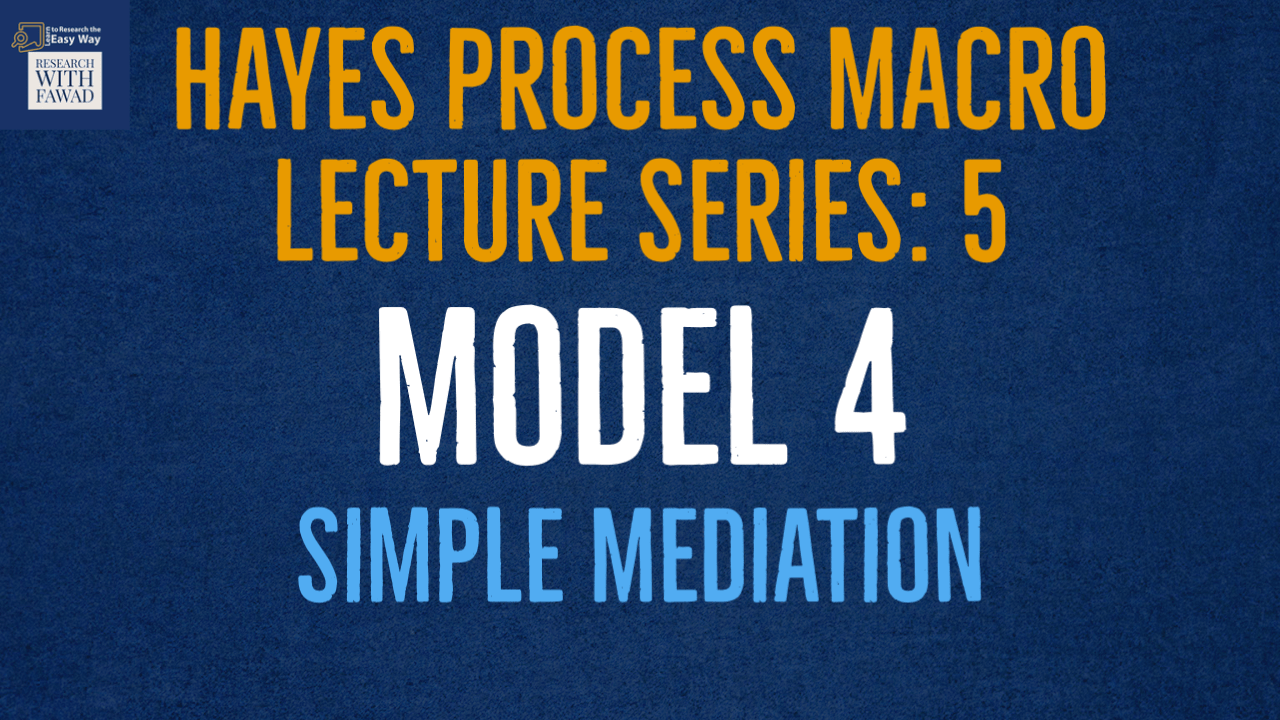### Hayes Process Model - Lecture Series

The tutorial will guide on Model 4 of the Hayes Process Macro for Mediation Analysis.

## Introduction

• Up to this point, we have focused on how to perform moderation analysis using the Process Macro.
• Let’s now examine how the influence between two constructs may take an indirect path through a third variable called a mediator.
• In these situations, the third variable will intervene on the influence of the two constructs (Hair et al. 2009).
• In testing if “mediation” or the presence of a mediator in a model, you need to understand some of the terminology that is used, such as direct effect, indirect effect, and total effects.
• A direct effect is simply a direct relationship between an independent variable and a dependent variable in presence of the Mediator (c’).

• An indirect effect is the relationship that flows from an independent variable to a mediator and then to a dependent variable (a*b).

• The term total effect is the combined influence of the direct effect between two constructs and the indirect effect flowing through the mediator (c = c’ + a*b).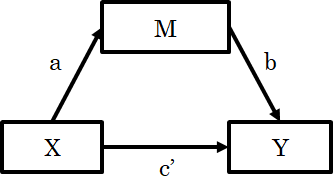• c in the relationship between X and Y is the total effect.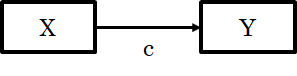## Example

• We want to examine if the construct of Culture has an indirect effect through Commitment on the construct of Organizational Performance.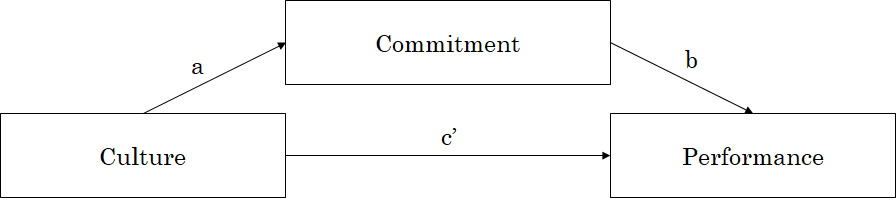## Conceptual and Statistical Diagram

• Example Variables: 1 predictor X, 1 Mediator M, and 1 Outcome Y
• The variables are continuous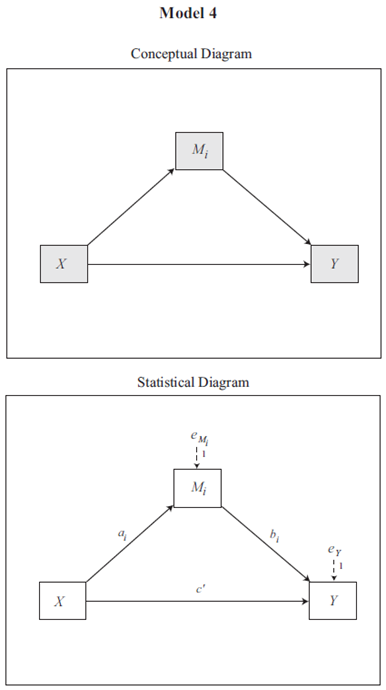## How to Run

• Step 1: Analyze -> Regression -> Process v4.0 by Andrew F. Hayes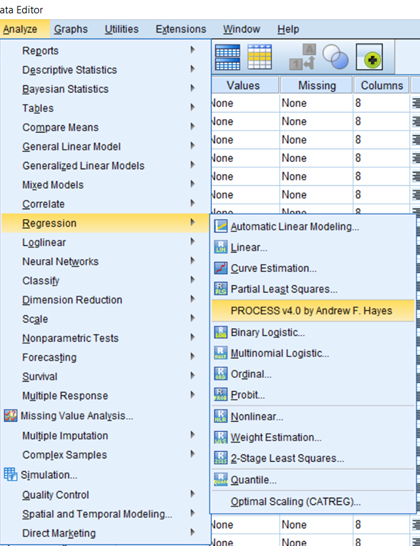• Step 2: Put in the Required Variables. In this case,
• Y Variable (Dependent Variable) is OP
• X Variable (Independent Variable) is Culture
• Mediator is Commitment
• Model Number is 4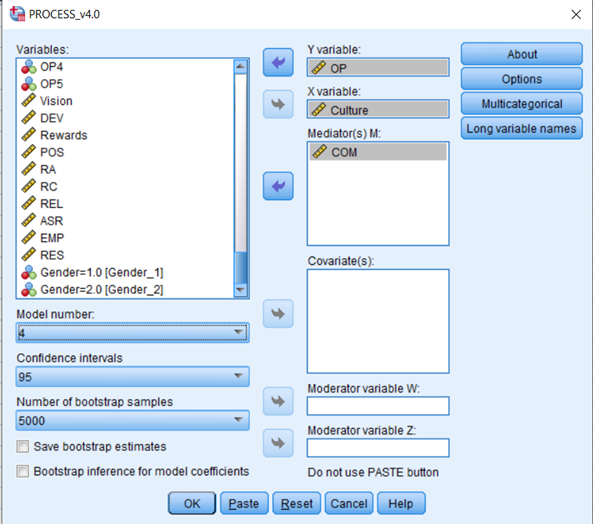• Step 3: Select Option Button, and Choose the options as shown in the figure below ()
• Select Show total effect model (only models 4,6,70,81, 82), this is to generate total effect estimates.
• For Standardized effect, select Standardized effects (mediation-only models).
• Press Continue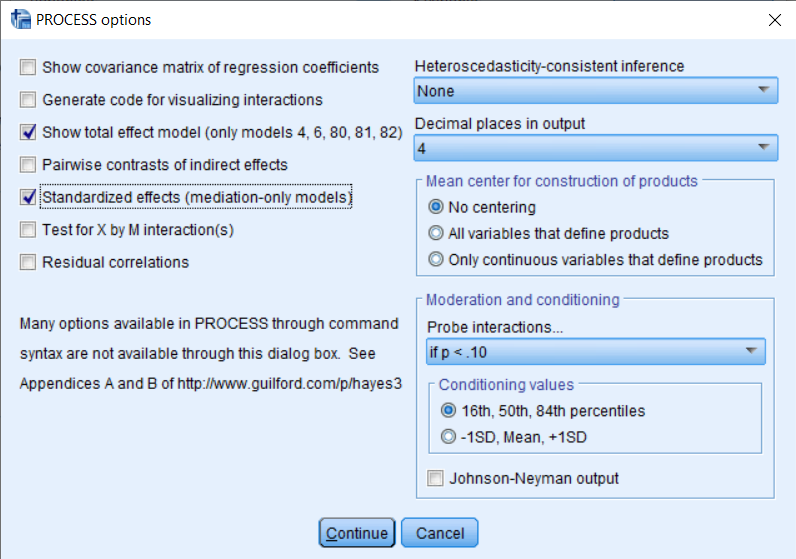## Output Interpretation - Basic Summary

• Description of Model along with the different variables where Y is Dependent Variable, X is Independent Variable, and M is the Mediating Variable.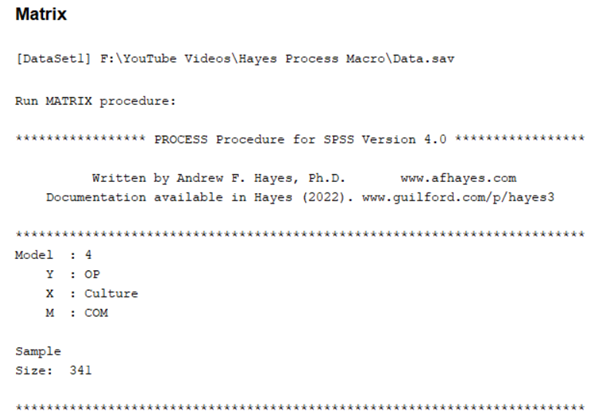## Output Interpretation - Model Summary

• Model Summary, provides summary of the model with R, R-Sq, F Statistics, and P value for the overall model.
• Next, is the coefficients, with impact of Culture on Commitment
• Culture has a significant impact on Commitment. This is path a.